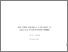# Some Recent Developments in the Theory and Computation of Linear Control Problems

Casti, J.L. (1975). Some Recent Developments in the Theory and Computation of Linear Control Problems. IIASA Research Memorandum. IIASA, Laxenburg, Austria: RM-75-053Preview
Text
RM-75-053.pdf

## Abstract

Recent analysis and computational results for the solution of linear dynamics-quadratic cost control processes are presented. It is shown that, if the number of system inputs and outputs is less than the number of state variables, a substantial reduction in computing effort may be achieved by utilizing the new equations, termed "generalized X-Y" functions over the standard matrix Riccati equation solution.

In addition to the basic X-Y equations, the paper also discusses the reduced algebraic equation for infin-infinite-interval problems, infinite-dimensional problems, the discrete-time case, and Kalman filtering problems. Numerical experiments are also reported.

Item Type: Monograph (IIASA Research Memorandum) System and Decision Sciences - Core (SDS) IIASA Import 15 Jan 2016 01:42 27 Aug 2021 17:08 http://pure.iiasa.ac.at/463View Item